# Algebra 1 : How to divide integers

## Example Questions

### Example Question #11 : Integer Operations

Divide: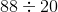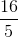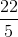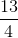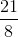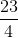Explanation:

Rewrite this expression into a fraction.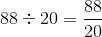Write the factors for the numerator and denominator.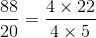The fours are common and can be divided or cancelled.

The result is:### Example Question #11 : Integer Operations

Divide: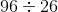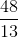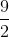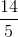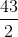Explanation:

In order to divide these two numbers, we will need to rewrite both numbers as a fraction and split the numbers by common factors.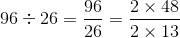The twos can be cancelled through division.

The answer is:### Example Question #2 : Division With Whole Numbers And Remainders

What is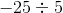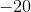Explanation:

When you are dealing with negative numbers, the following rules apply.

If there are no negative signs, the answer is positive.

If there is one negative sign, the answer is negative.

If there are two negative signs, the answer is positive.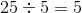, and since there is only one negative sign the answer is negative.is the solution.

### Example Question #13 : Integer Operations

Divide the following numbers: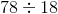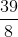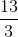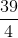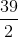Explanation:

Rewrite this expression as a fraction.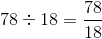Rewrite both numbers using common factors.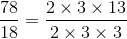Cancel the common terms in the numerator and denominator.

The answer is:### Example Question #14 : Integer Operations

Divide: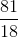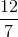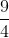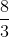Explanation:

Instead of using long division to solve, we can rewrite the numerator and denominator by their common factors.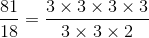Cancel the common terms in the numerator and denominator.

Write the remaining terms.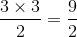The answer is:### Example Question #15 : Integer Operations

Divide the numbers: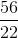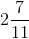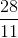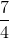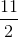Explanation:

Rewrite the numerator and denominator with common factors.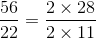Simplify the fraction.

The answer is:### Example Question #15 : Integer Operations

Divide the numbers: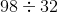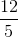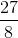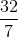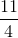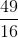Explanation:

Rewrite the expression into a fraction.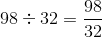Write the numbers in terms of their common factors.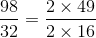Cancel the common terms.  There are no common factors with the numbers 49 and 16.

The answer is:### Example Question #1 : Order Of Operations And Pemdas

When evaluating the expression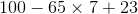,

in which order must you work the three operations?

Explanation:

By the order of operations, in the absence of grouping symbols, multplication must be worked before adding or subtracting. Then the addition and subtraction must be worked in left-to-right order; the subtraction is at left, so the subtraction is worked next, followed by the addition.

### Example Question #11 : Integer Operations

Divide twenty six by seventy eight.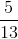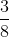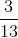Explanation:

Write the sentence as an expression.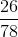Rewrite the fraction by common factors.  Both numbers are divisible by two.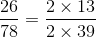Cancel out the two.

Notice that the fraction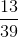can also be reduced further.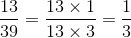The fraction is:### Example Question #12 : Integer Operations

Divide sixty with twenty five.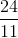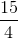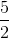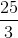Explanation:

Write the expression as a fraction.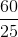Rewrite the numerator and denominator with common factors.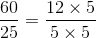Cancel the common terms on the top and bottom.

The answer is:### All Algebra 1 Resources